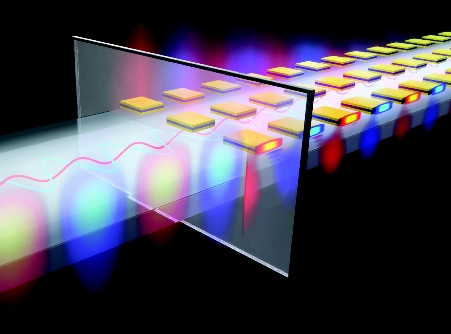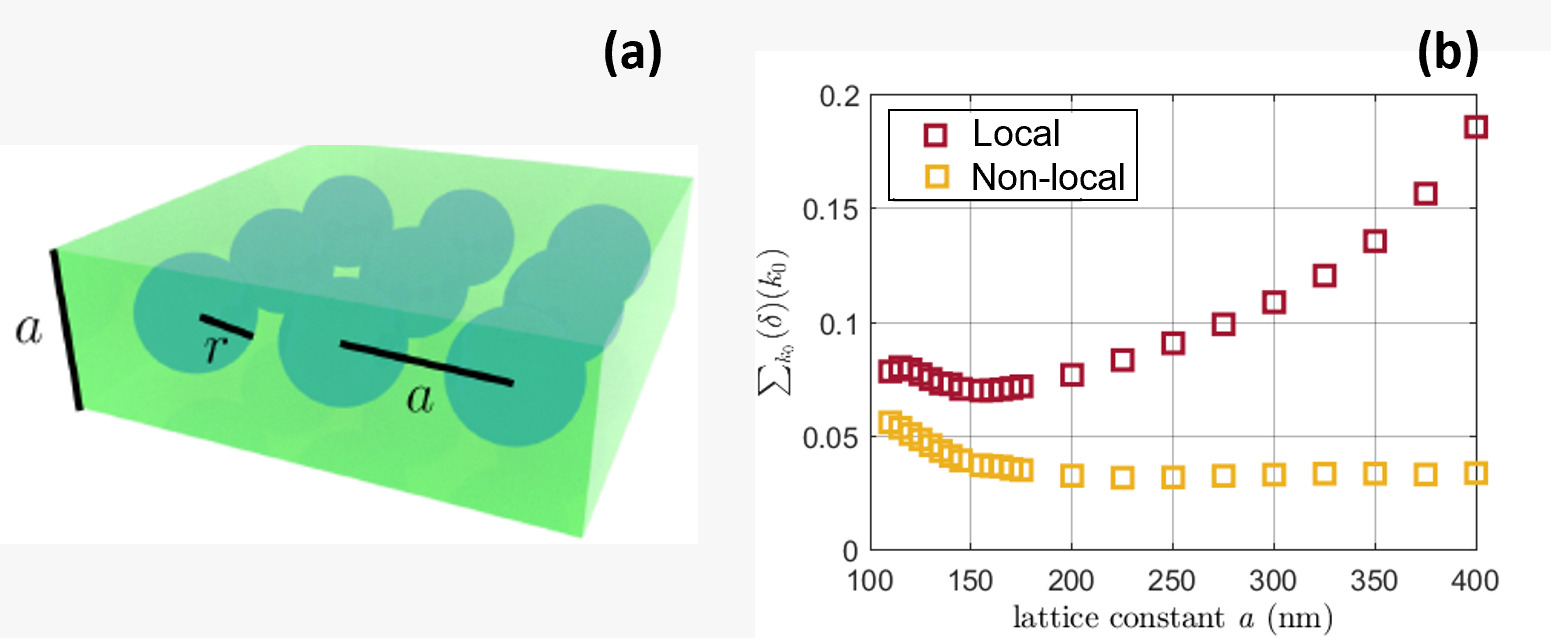# Project B4 • Effective characterization of optical metamaterials beyond a local response

### Principal investigators

 Prof. Dr. Michael Plum (7/2015 - ) Prof. Dr. Carsten Rockstuhl (7/2015 - )

### Project summary

In this project, we aim to improve the description of light-matter interaction in optical metamaterials by describing them at the effective level with nonlocal constitutive relations. To this end, we formulate these constitutive relations, study elementary solutions to Maxwell's equations in these materials and explore their dispersion relation, rigorously derive (additional) interface conditions to study finite structures, and homogenize specific metamaterials and explore physical aspects of these nonlocal constitutive relations.Figure 1. Illustration of an interface between vacuum and an optical metamaterial made of cut-plate pairs.

#### Introduction and methodology

Optical metamaterials are made of periodically arranged sub-wavelength inclusions called meta-atoms. These materials show interesting effects that cannot be observed in natural media, e.g., a negative refraction index or negative magnetic permeability. To discuss these effects on physical grounds, it is a prime challenge to introduce and assign effective material parameters to a given metamaterial by assuming that their corresponding parameters are spatially independent. This step is called homogenization.

Local, effective material parameters are frequently employed to describe metamaterials at the effective level. Still, these are insufficient to describe many actual metamaterials in the operational wavelength regime. Therefore, when an affective description with local constitutive relation is no longer possible, the metamaterial is said to be spatially dispersive or nonlocal.

Our project aims to go beyond this local description and to work out all aspects for an accurate description of the spatial dispersion of actual metamaterials. This can be seen by answering the question: how can we improve the effective description of optical metamaterials such that the macroscopic properties of the material are correctly captured?

It is noteworthy that when the ratio of the size of the unit cell to the wavelength tends to be in the order of one (but still smaller), spatial variations of the fields across the unit cell tend to be significant. In that size regime, the light tends to see'' the fine geometry of the unit cell. Therefore, introducing nonlocal effective material properties is of utmost importance, as the local description is inadequate in this regime. Nonlocality can be modelled as follows \begin{equation} \mathbf{D}(\mathbf{r}, \omega) = \int_{\mathbb{R}^3} \mathbf{R}(\mathbf{r} - \mathbf{r}\prime , \omega) \mathbf{E} (\mathbf{r}\prime , \omega) d\mathbf{r}. \end{equation} This relation is understood as follows. The electromagnetic response described by the electric displacement $\mathbf{D}$ at a position $\mathbf{r}$ is induced by an electric field $\mathbf{E}$ at a distant position $\mathbf{r}\prime$. This response is mediated by the response function $\mathbf{R}$ that depends on both positions. Since the material is homogeneous, in the response function only $\mathbf{R}$ depends on the relative positions of $\mathbf{r}$ and $\mathbf{r}\prime$, i.e., the difference $\mathbf{r}-\mathbf{r}\prime$. In that equation, all quantities depend on the frequency $\omega$.

It is true that at the effective level, the response $\mathbf{R}$ gives the exact description of the homogenized metamaterial. But we don't have an exact formula for it. Therefore, one major task in this project consists of writing admissible representations of the nonlocal response functions $\mathbf{R}$ by means of approximation theory.

One approach was to consider the following Taylor approximation \begin{equation} \widehat{\mathbf{R}}(\mathbf{k},\omega)=\varepsilon +\mathbf{k} \times \alpha \mathbf{k} \times - \mathbf{k} \times \mathbf{k} \times \gamma \mathbf{k} \times \mathbf{k} \times, \end{equation} where $\varepsilon$, $\alpha$ and $\gamma$ are $3\times3$ matrices depending on $\omega$ and represent the effective material parameters. By inverse Fourier transform we can remount to differential operators. This formulation helps to derive interface conditions through the weak formulation of the obtained model.

Alternatively, considering rational approximation for the response function, written \begin{equation} \widehat{\mathbf{R}}(\mathbf{k},\omega)= \left[ I_{3} - \widehat{\mathbf{q}} (\mathbf{k},\omega)\right]^{-1} \left[ \varepsilon + \widehat{\mathbf{p}} (\mathbf{k},\omega)\right], \end{equation} where $I_{3}$ refers to the identity matrix, $\widehat{\mathbf{p}}(\cdot,\omega)$ and $\widehat{\mathbf{q}}(\cdot,\omega)$ are $\mathbb{C}^{3\times 3}$ matrices, polynomially depending on $\mathbf{k}$ and vanish at $\mathbf{k} = 0$. Different choices for the polynomials $\mathbf{p}$ and $\mathbf{q}$ allow deriving different characterizations for the approximate nonlocal material law. Another concern is studying these formulations and establishing a checklist allowing us to analyze all the obtained wavelike equations. This can be done in particular by writing the interface conditions explicitly for each model. Further, we solve the bulk problems by computing and solving the dispersion relations which are required to define the propagating eigenmodes within a metamaterial.

To have a more accurate representation of the response function, we proceed by retaining higher-order terms in the polynomial approximation. A challenging task is to compute the additional interface condition at any order in compact formulas. This is done so by invoking the weak formulation of the obtained model.

#### Results and perspective

During our first funding period, we dedicated ourselves to finding sensible mathematical models to redefine effective medium theory descriptions by incorporating nonlocality in the effective description of metamaterials. We also established suitable tools to solve the Maxwell equations with nonlocal constitutive relations characterized by $\varepsilon,~\mu, \text{and}~\gamma$. The tools could calculate the propagating modes inside the metamaterial volume and reflection and transmission coefficients at metamaterial boundaries and entire slabs.

In the second funding period, we took it upon ourselves to study the physical intuitions offered by these additional material parameters and thereby understand the limits of our homogenization model. Here we discuss one such result. In Fig. 1 (a), we show infinite copies of an electric dipole sphere of radius $r$ arranged in an array with a lattice constant (distance between the consecutive spheres) $a$. In this study, we systematically judge the possibility of homogenizing the different configurations of this array by sweeping over $a$. Decreasing $a$ corresponds to a denser packing fraction, invoking strong inter-particle interactions among the spheres. To judge the quality of the homogenization, we define a mean error function $(\delta)$ summed over the considered spectrum $k_0$. Our assessment goes by as, the smaller the value of $\delta$, the less erroneous the predictions of the optical properties are by the homogenization model. For comparison, we show here the results from both the nonlocal and the conventional local form of the constitutive relation.Firstly, the SSD model is always superior to the WSD model. The error for the SSD model is always smaller than that for the WSD model. This improvement is especially convincing towards the larger $a$ where the SSD model additionally shows stability. These improvements are attributed to the nonlocal $\gamma$ parameter that accounts for the strong interactions among the spheres.

Secondly, toward the shorter lattice constants, beyond $a = 200 nm$ the error function for both the SSD and the WSD models begins to grow. This suggests a critical value, $a_{\text{critical}}$ below which homogenization is not feasible anymore. This is because for $a < a_{\text{critical}}$ the adjacent spheres are almost touching, therefore, resulting in a spread of excitation across the material by means of strong near-field interactions. This nature limits the applicability of the homogenization model for $a < a_{\text{critical}}$ and therefore is the lower limit for the homogenization of the considered array.

Therefore, there exists an optimal period over which homogenization is feasible. This insight can be safely expected to shape our understanding of how to homogenize any metamaterial.

## Publications

1. , , , , , , and . A T-matrix based approach to homogenize artificial materials. Adv. Opt. Mater., 11(3):2201564, February . [bibtex]

2. , , , , , and . Modeling four-dimensional metamaterials: a T-matrix approach to describe time-varying metasurfaces. Opt. Express, 30(25):45832–45847, December . [preprint] [files] [bibtex]

3. , , , , , , , , , , and . Floquet–Mie theory for time-varying dispersive spheres. Laser Photonics Rev., 2100683, December . Online first. [bibtex]

4. , , and . Artificial neural networks used to retrieve effective properties of metamaterials. Opt. Express, 29(22):36072–36085, October . [bibtex]

5. , , , , and . Higher order constitutive relations and interface conditions for metamaterials with strong spatial dispersion. Phys. Let. A, 412(19):127570, October . [preprint] [bibtex]

6. , , , , , and . Lower limits for the homogenization of periodic metamaterials made from electric dipolar scatterers. Phys. Rev. B, 103(19):195425, May . [preprint] [bibtex]

7. , , , , and . Towards more general constitutive relations for metamaterials: a checklist for consistent formulations. Phys. Rev. B, 101(19):195411, May . [preprint] [bibtex]

8. , , , and . Homogenization of wire media with a general purpose nonlocal constitutive relation. J. Opt. Soc. Am. B, 36(8):F99–F108, August . [bibtex]

9. , , , and . Retrieving effective material parameters of metamaterials characterized by nonlocal constitutive relations. Phys. Rev. B, 99(3):035442, January . [bibtex]

10. , , and . Exact multipolar decompositions with applications in nanophotonics. Adv. Opt. Mater., 7(1):1800783, January . [bibtex]

11. , , , , , and . Surface plasmon polaritons sustained at the interface of a nonlocal metamaterial. Phys. Rev. B, 98(11):115409, September . [bibtex]

12. , , , , and . Beyond local effective material properties for metamaterials. Phys. Rev. B, 97(7):075439, February . [preprint] [bibtex]

13. and . Spectrum of a singularly perturbed periodic thin waveguide. J. Math. Anal. Appl., 454(2):673–694, October . [preprint] [bibtex]

14. , , , and . Computing the T-matrix of a scattering object with multiple plane wave illuminations. Beilstein J. Nanotechnol., 8:614–626, March . [bibtex]

15. , , , and . Transverse multipolar light-matter couplings in evanescent waves. Phys. Rev. A, 94(5):053822, November . [preprint] [bibtex]

16. , , , and . Optically assisted trapping with high-permittivity dielectric rings: Towards optical aerosol filtration. Appl. Phys. Lett., 109(14):141102, October . [preprint] [bibtex]

17. , , , and . Exact dipolar moments of a localized electric current distribution. Opt. Express, 23(26):33044–33064, December . [preprint] [bibtex]

## Preprints

1. and . Approximate mechanical impedance of a thin linear elastic slab. CRC 1173 Preprint 2022/31, Karlsruhe Institute of Technology, July . [bibtex]

2. , , , , and . Interface conditions for a metamaterial with strong spatial dispersion. CRC 1173 Preprint 2017/23, Karlsruhe Institute of Technology, September . [bibtex]

## Theses

1. . Modeling optical metamaterials with strong spatial dispersion. PhD thesis, Karlsruhe Institute of Technology (KIT), November . [bibtex]

2. . Spectral and asymptotic properties of periodic media. Habilitation thesis, Karlsruhe Institute of Technology (KIT), January . [bibtex]

Former staff
Name Title Function
Dr. Postdoctoral researcher
Dr. Postdoctoral researcher
Dr. Doctoral researcher
Dr. Postdoctoral researcher
Dr. Postdoctoral researcher